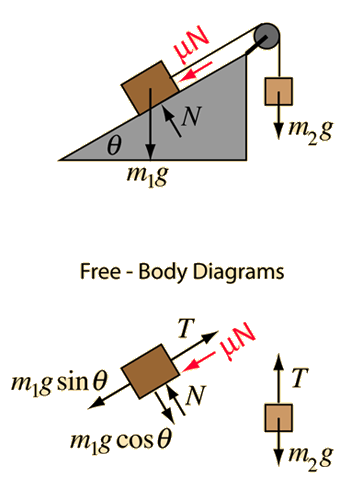# Inclined Pulley with FrictionNote that the tension in the rope is NOT equal to the weight of the hanging mass except in the special case of zero acceleration.

Friction must be treated with care in a situation like this. If the hanging mass is large enough to overcome friction and accelerate the mass upward on the incline, then the friction force will oppose it and act downward. If the mass on the incline is large enough, it will overcome friction and move downward, pulling the hanging mass upward. In this case the friction force will act up the incline. There is an intermediate range of masses where the block will move neither up nor down the incline.

Application of Newton's second law to mass on incline with pulley.
Given an incline with angle degrees which has a mass of kg placed upon it. It is attached by a rope over a pulley to a mass of kg which hangs vertically. The friction between the mass and the incline is represented by a friction coefficient mu= . Taking downward as the positive direction for the hanging mass, the acceleration will be

### Acceleration =m/s²

With this acceleration, the tension in the rope will be
T= Newtons compared to the weight W = Newtons for the hanging mass. Exploring different values for the masses will allow you to show that the tension is less than the weight for downward accelerations and greater than the weight for upward accelerations when the net force on the hanging mass must be upward.

By changing masses, you can explore whether the mass will move up the incline, downward, or remain at rest. This calculation tests whether the masses are sufficiently different to overcome friction in either direction. If the resulting acceleration is zero, that indicates that the imbalance of forces is not sufficient to overcome friction in either direction.

### Expressions****Remove friction

Index

Newton's laws

Standard mechanics problems

 HyperPhysics***** Mechanics ***** Newton's laws R Nave
Go Back